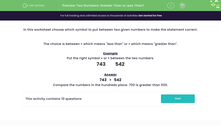# Two Numbers: Greater Than or Less Than?

In this worksheet, students must place either the < symbol or the > symbol between two given numbers to make the statement correct.Key stage:  KS 2

Curriculum topic:   Number: Number and Place Value

Curriculum subtopic:   Order/Compare Numbers to 1000

Difficulty level:#### Worksheet Overview

In this worksheet choose which symbol to put between two given numbers to make the statement correct.

The choice is between < which means "less than" or > which means "greater than".

Example

Put the right symbol > or < between the two numbers.

743 542